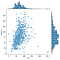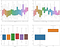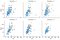# Python Series 1: Visualizing Data for Beginners Using Seaborn

by: Robby Alfardo Irfan, Learner by doing

Hi there, this is me with my first project about Explanatory Data Analysis (EDA). It is an approach for data analysis that employs a variety of techniques (mostly graphical). It is used to discover patterns, spot anomalies, check assumptions or test a hypothesis through summary statistics and graphical representations.

In this series, we will learn how to perform EDA using data visualization. Particularly, focus on `seaborn`, a Python data visualization library based on `matplotlib` and integrates closely with `pandas` pandas data structures.

Seaborn packages helps you explore and understand your data. Its plotting functions operate on data frames and arrays containing whole datasets and internally perform the necessary semantic mapping and statistical aggregation to produce informative plots.

• Numerical variables with histograms,
• Categorical variables with count plots and pie chart,
• Relationships between numerical variables with scatter plots, joint plots, and pair plots, and
• Relationships between numerical and categorical variables with box-and-whisker plots and complex conditional plots

Okay, let’s get this show on the road!!!

# Data Preparation

Data preparation is the first step of any data analysis to ensure data is cleaned and transformed in a form that can be analyzed. We will be performing EDA on the Myles O’Neill dataset about the pokemon games (NOT pokemon cards or Pokemon Go).

This dataset is already cleaned and ready for analysis. Let’s begin by reading our data as a `pandas` DataFrame:

`import pandas as pdimport matplotlib as pltpokemon = pd.read_csv('.../input/pokemon.csv')pokemon.info()pokemon.head()`

If you run this code in a Jupyter notebook or in a Spyder, you can see that there are 800 observations and 12 columns. Each column represents a variable in the DataFrame. We can see from the data type of each column what type of variable it is. Let’s move onto some analysis!

# Analyzing Numerical Variables

Numerical variables are simply those for which the values are numbers. The first thing that we do when we have numerical variables is to understand what values the variable can take, as well as the distribution and dispersion. This can be achieved with a histogram:

`import seaborn as snssns.set(style='whitegrid', palette="deep", font_scale=1.1, rc={"figure.figsize": [8, 5]})sns.distplot(pokemon['Total'], norm_hist=False, kde=False, bins=20, hist_kws={"alpha": 1}).set(xlabel='Total Power', ylabel='Count')`

We are able to style our figure, change the color, increase the font size for readability, and change the figure size using `sns.set()` .

We use `distplot` plot histograms in `seaborn`. This by default plots a histogram with a kernel density estimation (KDE). You can try changing the parameter `kde=True` to see what this looks like.

Taking a look at the histogram, the distribution has two peaks. Most pokemon have total power between 300 and 350 and between 450 and 550.

Pandas package offers the simplest solution to create histograms for all of our numerical variables. Here is the solution:

`pokemon[['Total','HP','Attack','Defense','Sp. Atk','Sp. Def','Speed']].hist(bins=15, figsize=(15, 6), layout=(2, 4))`

We can get all pieces of information from this visualization. We can see that `HP` ,`Attack` ,`Defense` ,`Sp. Atk` ,`Sp.Def` are heavily skewed right. While `Total` has a bimodal distribution and `Speed` looks like a normal distribution.

Note that the visualizations keep the style that we set previously using `seaborn`.

# Analyzing Categorical Variables

Categorical variables are those for which the values are labeled categories. The values, distribution, and dispersion of categorical variables are best understood with bar plots or to know the percentage is best understood with pie charts.

`chart = sns.countplot(pokemon['Type 1'])chart.set_xticklabels(chart.get_xticklabels(), rotation=30)`

From the chart, we can see that `Water` pokemon dominate the pokemon types 1 and `Flying` pokemon are the fewest.

We can also visualize all the categorical variables in our dataset, as we did with the numerical variables. We can loop through `pandas` series to create subplots.

`fig, ax = plt.subplots(1, 4, figsize=(40, 10))for variable, subplot in zip(pokemon[['Type 1', 'Type 2','Generation','Legendary']], ax.flatten()):    sns.countplot(pokemon[variable], ax=subplot)    for label in subplot.get_xticklabels():        label.set_rotation(90)`

As with our numerical variable histograms, we can gather lots of information from this visual. We can see that `Flying` pokemon become the most of the pokemon types 2 and `Bug` pokemon are the fewest. Also, we can see that the number of odd generations of pokemon is always more than the number of even generations of pokemon. Lastly, `Legendary` pokemon are just a few rather than `Normal` pokemon.

We can also use a pie chart to visualize both the categorical variable and numerical variable. However, we only visualize the categorical one. Pie charts are used effectively when using just a few classes of variables because they will be easier to be seen and understood. Also, when we focus on the percentage more, pie charts will be the recommended charts. Here is the example of a pie chart:

`from matplotlib import pyplot as pltimport numpy as npfig = plt.figure()ax = fig.add_axes([0,0,1,1])ax.axis('equal')langs = pokemon['Legendary'].value_counts().indexsizes = pokemon['Legendary'].value_counts().valuesax.pie(sizes, labels = langs,autopct='%1.2f%%')plt.show()`

In this chart, we can see directly that the proportion of `Legendary` pokemon are very few.

We have explored our numerical and categorical variables, let’s take a look at the relationship between these variables!

# Analyzing Relationships Between Numerical Variables

Plotting relationships between variables allows us to examine whether or not there is a relationship (association) between the variables plotted.

Scatter plots are used to plot data points on a horizontal and a vertical axis in the attempt to show how much one variable is affected by another. The scatter plot is often used for visualizing relationships between two numerical variables. The `seaborn` method to create a scatter plot is very simple:

`sns.scatterplot(x=pokemon['HP'], y=pokemon['Attack'])`

From the scatter plot, we see here that we have a positive relationship between the `HP` pokemon and the `Attack` of the pokemon. In other words, the larger the HP of the pokemon, the higher the likely attack of the pokemon. Even though, there some outliers that have `HP` more with the less `Attack` .

`seaborn` also provides us with a nice function called `jointplot` which will give you a scatter plot showing the relationship between two variables along with histograms of each variable in the margin. It is also known as a marginal plot.Jointplot showing relationship between HP and Attack and their individual distributions.

Not only we can see the relationships between the two variables, but also how they are distributed individually.

# Analyzing Relationships Between Numerical and Categorical Variables

In order to visualize relationships between numerical variables and categorical variables, we commonly use the box-and-whisker plot. In addition, to visualize conditional relationships, we can use complex conditional plots.

Let’s get started by creating box-and-whisker plots with `seaborn`’s `boxplot` method:

`fig, ax = plt.subplots(2, 2, figsize=(25, 20))for var, subplot in zip(pokemon[['Type 1', 'Type 2','Generation','Legendary']], ax.flatten()):    sns.boxplot(x=var, y='Total', data=pokemon, ax=subplot)`Box-and-whisker plots for each of our categorical variables and their relationships with pokemon’s total power

Here, we have iterated through every subplot to produce the visualization between all categorical variables and the `Total`.

We can see that `Dragon` pokemon even as type 1 or type 2 have the highest average `Total` power than the others. Also, we can see that pokemon has the almost same range and average in every `Generation`. `Legendary` pokemon have a totally different range and average rather than `Normal` pokemon.

Finally, `seaborn` also allows us to create plots that show conditional relationships. For example, if we are conditioning on the `Generation`, using the `FacetGrid` function we can visualize a scatter plot between the `HP` and the `Attack` variables with `Legendary` class:

`cond_plot = sns.FacetGrid(data=pokemon, col='Generation', hue='Legendary', col_wrap=3)cond_plot.map(sns.scatterplot, 'HP', 'Attack')`The conditional plot between HP and attack to generation with legendary class.

For each generation, we can see the relationship between `HP` and `Attack`.

We also added another categorical variable `Legendary` to the (optional) `hue` parameter, the orange points correspond to `Normal` pokemon. As you can see, all pokemon’s generations have a positive relationship between the `HP` pokemon and the `Attack` of the pokemon. But the third and fourth generations are mostly blended between `Legendary` and `Normal` pokemon.

The `FacetGrid` method makes it incredibly easy to produce complex visualizations and to get valuable information. It is good practice to produce these visualizations to get quick insights into variable relationships.

I hope you’ve enjoyed this brief tutorial on exploratory data analysis and data visualization with `seaborn`! We covered how to create histograms, count plots, pie charts, scatter plots, marginal plots, box-and-whisker plots, and conditional plots. See ya in the next series!!!